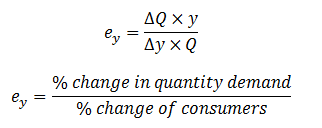## TyroCity

Economics 12 Notes for Economics Notes

Posted on • Updated on

# Income Elasticity of demand

It refers to the percentage change in quantity demand due to the certain percentage change in income of consumers when other things remaining the same.

Mathematically, it can be expressed as:Where,
ey = income elastic of demand
∆Q = change in quantity demand (i.e. Q2 – Q1)
∆y = change in income
y = initial income
Q = initial quantity demand